Mechanics Competition by shubham dhull

Welcome all to the Brilliant Mechanics Contest. Like the Brilliant Integration Contest, the aim of the Mechanics Contest is to improve skills and techniques often used in Olympiad/JEE-style Mechanics problems. But above all, the main reason is so we can have fun.

Anyone is allowed to participate, as long as they adhere to the following rules.

I will post the first problem. If someone solves it, he or she can post a solution and then must post a new problem.

A solution must be posted below the thread of the problem. Then, the solver must post a new problem as a separate thread.

Please make a substantial comment.

Make sure you know how to solve your own problem before posting it, in case no one else is able to solve it within 48 hours. Then, you must post the solution and you have the right to post a new problem.

If the one who solves the last problem does not post a new problem in 24 hours, the creator of the previous problem has the right to post another problem.

The scope of the problems is (IPhO) Olympiad Mechanics.

Keep yourself restricted to Newtonian Mechanics. Do not use Lagrangian mechanics

You are allowed to apply a slight dressing of Electricity And Magnetism to your Mechanics Problem.

Upload your solution along with the answer * Compulsory. *

It is NOT compulsory to post original problems. But make sure it has not been posted on brilliant.

Try to post the* simplest solution* possible. For example, if someone posted a solution using Newton's Laws, when there is a solution using only Work Energy theorem, the latter is preferred.

There should be only one question. No sub questions allowed.No parts. Only one answer should be there.

The problem creator should not give the answer to his/her question with the question.

Post only the problem and it's solution here. Extremely relevant comments are allowed. All discussions should strictly not be done here.Note by A Former Brilliant Member
3 years ago

This discussion board is a place to discuss our Daily Challenges and the math and science related to those challenges. Explanations are more than just a solution — they should explain the steps and thinking strategies that you used to obtain the solution. Comments should further the discussion of math and science.

When posting on Brilliant:

• Use the emojis to react to an explanation, whether you're congratulating a job well done , or just really confused .
• Ask specific questions about the challenge or the steps in somebody's explanation. Well-posed questions can add a lot to the discussion, but posting "I don't understand!" doesn't help anyone.
• Try to contribute something new to the discussion, whether it is an extension, generalization or other idea related to the challenge.
• Stay on topic — we're all here to learn more about math and science, not to hear about your favorite get-rich-quick scheme or current world events.

MarkdownAppears as
*italics* or _italics_ italics
**bold** or __bold__ bold

- bulleted
- list

• bulleted
• list

1. numbered
2. list

1. numbered
2. list
Note: you must add a full line of space before and after lists for them to show up correctly
paragraph 1

paragraph 2

paragraph 1

paragraph 2

> This is a quote
This is a quote
# I indented these lines
# 4 spaces, and now they show
# up as a code block.

print "hello world"
# I indented these lines
# 4 spaces, and now they show
# up as a code block.

print "hello world"
MathAppears as
Remember to wrap math in $$...$$ or $...$ to ensure proper formatting.
2 \times 3 $2 \times 3$
2^{34} $2^{34}$
a_{i-1} $a_{i-1}$
\frac{2}{3} $\frac{2}{3}$
\sqrt{2} $\sqrt{2}$
\sum_{i=1}^3 $\sum_{i=1}^3$
\sin \theta $\sin \theta$
\boxed{123} $\boxed{123}$

Sort by:

It should be r instead of R

- 2 years, 3 months ago

Post any "good" mechanics or physics question you come across your preparation, I'll also post if I come across any.

Thanks.

- 3 years ago

Problem 6 SET I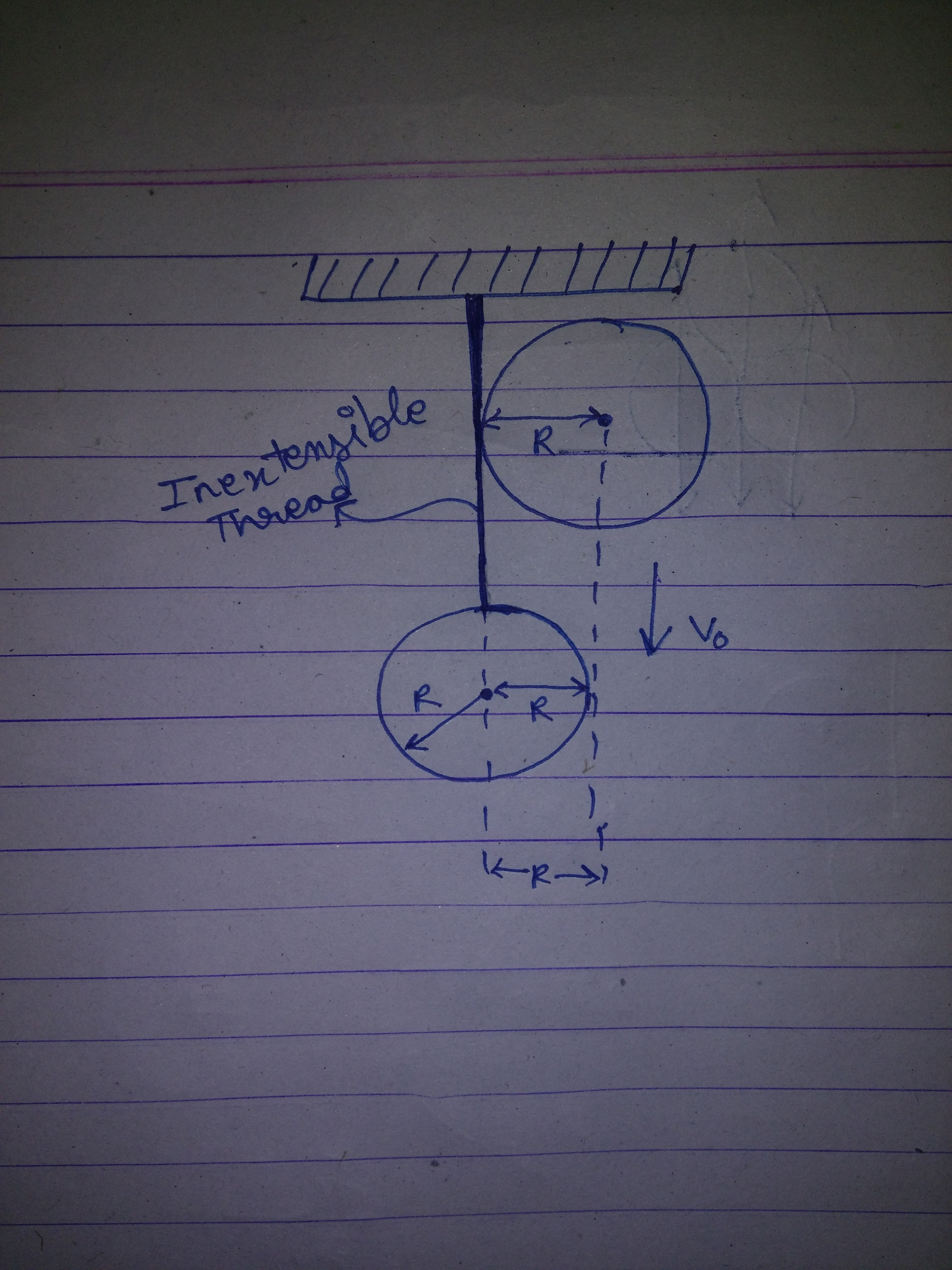A Bob of mass m is hanging by an inextensible thread. A similar of Bob of mass m and same radius is going to strike the Bob held with string as shown in the figure. It strikes the the Bob with a speed of $v_{0}$.

Find the velocities of both the bobs after the collison.

- 3 years ago

Correct??

- 3 years ago

Lower bob's horizontal velocity $v = 2\sqrt{3}/5$

Upper bob's after collision velocity $v2 = \sqrt{13}/5$

- 3 years ago

bro, solution? rule here

You only told , no rules from now , and only we 3 are there , is their a need for solution??

- 3 years ago

Correct!

Anybody want to post a question?

- 3 years ago

Somebody solvey optics q , it had got more than 180 views , but 0 solvers ! :P

- 3 years ago

i will try after phase test :P and after net also gets unlimited

- 3 years ago

I can't solve it, havent studied it yet.

- 3 years ago

i'll try

i'll post

COOL!!!

- 3 years ago

ok, no i dob't need the sol. , i can do it thnx

yes tell wheter elastic or not

Is e given??

- 3 years ago

Sorry, forgot to mention that e=1:P

- 3 years ago

Try it guyz!

- 3 years ago

I won't try , otherwise I would be forced to post the next q :P ( And I lack time )

Prakhar also won't be online for 2 weeks . Furthermore this competition lacks participation , it is not arousing interest in me ...

- 3 years ago

Will you post questions?

- 3 years ago

Whenever I will get time :)

By that time you can check my Brilliant questions ( I posted) if you want :)

- 3 years ago

K, we have just started rotational mechanics in Fiitjee, so after that, I'll become eligible to try mechanics questions on brilliant!

- 3 years ago

OK, what answer are you getting?

- 3 years ago

No problem.

The new brilliant interface has made it difficult to discover "new" stuffs.

@Calvin Lin please do something, the community is going to a hibernation due to new boring interface, the new interface is not at all logical.

- 3 years ago

Problem 4. SET I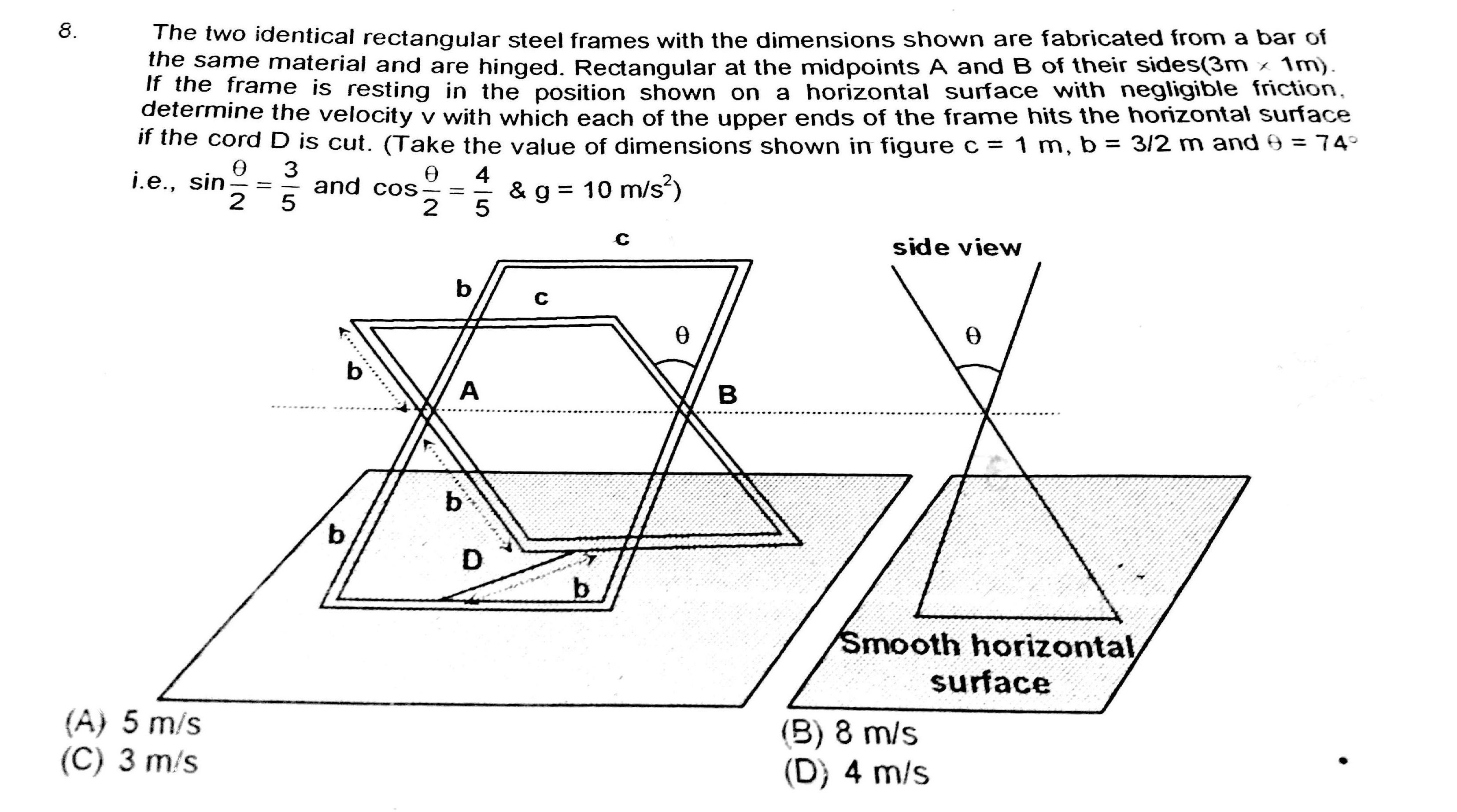- 3 years ago

hey @Aniket Sanghi is the answer 8 ?

if my answer is correct, let me share the solution ! let the mass of the rods be m1 , m2 ( it would help if u assume some mass per unit length ) the B will move downward with some velocity v , the line bb will only have a horizontal velocity by using geometry u can fid the relations in their velocities and then using conservation of energy , we will get the velocity as 8 m/sec. easy enough !@Aniket Sanghi @Prakhar Bindal bhai !

Answer is correct ! 👍 Post the next!

- 3 years ago

ok here is it $set-1$ $q-5$ An easy one A thin uniform equilateral triangular plate rests in a vertical plane with one of its ends (B) on a rough horizontal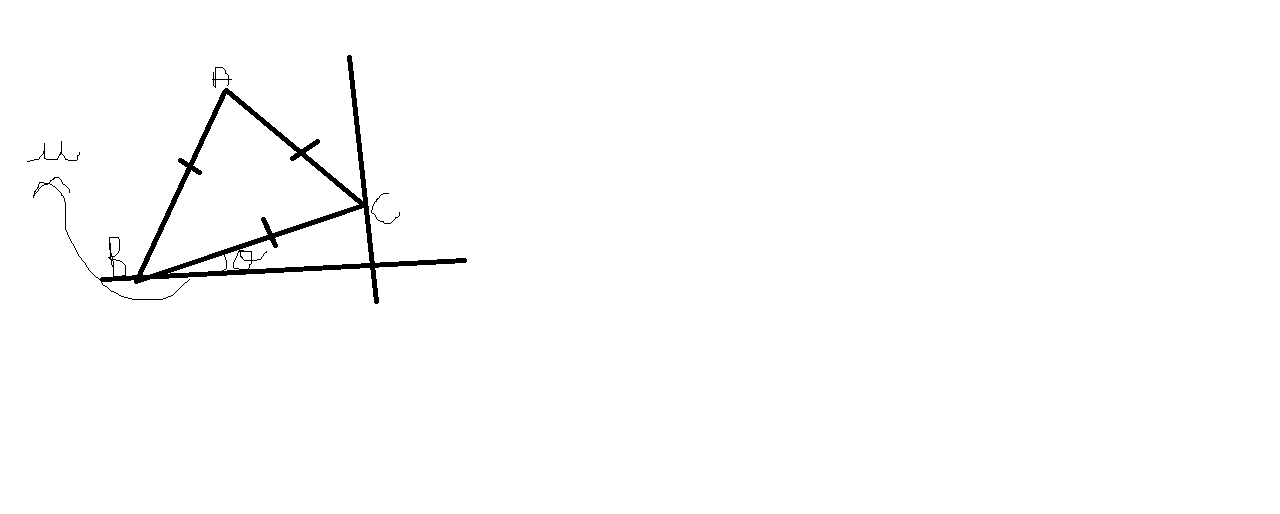floor and the other end (C) on a smooth vertical wall. The least angle its base (BC) can make with horizontal will be:

Am I correct ?

- 3 years ago

YES YOU'RE RIGHT ! also i liked it that u uploaded the question without uch delay , good work !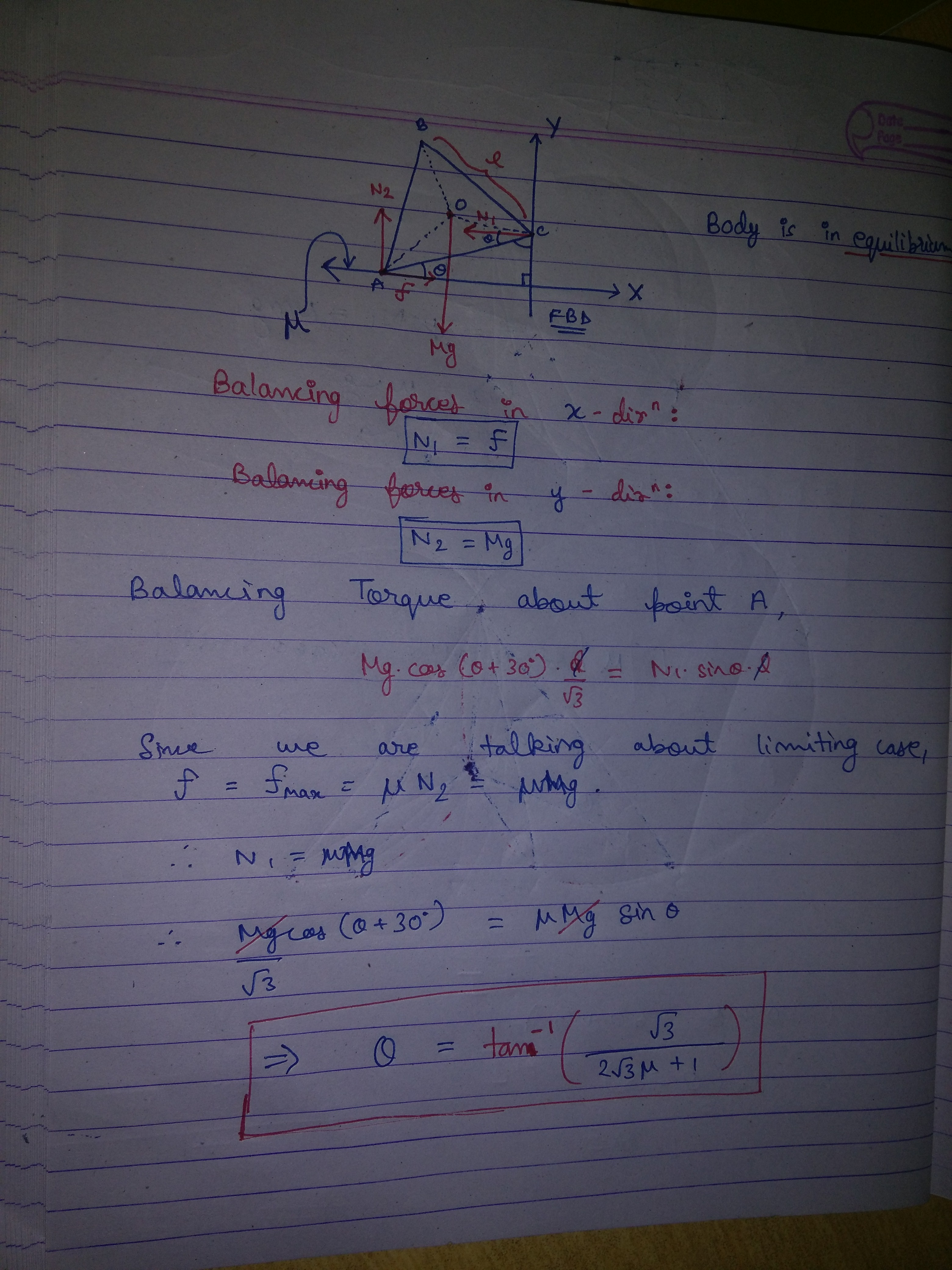- 3 years ago

Why is the contest stubbed?

- 3 years ago

An uniform rope of mass m is resting on a sphere such that it covers a circle of radius r on the sphere.

The radius is sphere is R.

Find the tension in the rope.

- 3 years ago

Take a small element in the rope dx (mass = dm =$\lamda R (2d\theta)$ )

Check the normal reaction and divide it in components in horizontal and vertical direction and we get

$N cos\theta = dm g$

$N sin\theta = 2 T sin (d\theta)$

On simplifying we get

$\boxed{ T = \frac {mgR}{2\pi \sqrt {R^2 - r^2}}}$

- 3 years ago

It should be r instead of R

- 2 years, 3 months ago

Correct!

Post the next question.

- 3 years ago

hey bro whats the situation can you explain or you @Harsh Shrivastava . if possible please attach a diagram

- 3 years ago

A rope is kept surroundimg a sphere of radius R, the circumference of rope is less than 2piR.

- 3 years ago

Set(1) Problem 2

A Shell flying with velocity v= 500 m/sec bursts into three identical fragments so that kinetic energy of system increases 1.5 times. Maximise the velocity that one of the fragments can obtain.

this is an integer type problem (0 to 9)

- 3 years ago

It is more a maths problem than physics.

Let the velocities of the fragment be x,y,z after the explosion.

Conserving momentum along initial direction of motion, 500m = m/3 (x+y+z).

Also, using the KE data, x^2 + y^2 + z^2 = 1125000.

Using these two equations, we can say :

$\dfrac{(1500-x)^2}{2} \leq 1125000-x^2$

Thus we get -1000m/s <= x<= 0m/s

- 3 years ago

hey, post the next question !

Right! . post the next.

Did you had your first AIITS? . What was ur rank?

- 3 years ago

Very bad, 126 :(

- 3 years ago

plz move such discussions to slack or other notes and delete ur comment here ! it's only for asking questions not talking !!

Ohk sorry

- 3 years ago

- 3 years ago

Some more Invitations

- 3 years ago

I am starting a second set of question series (Advance Level / IPho Level) , as when 1st serious take a break the contest must go on! Both series will go independently . I hope @shubham dhull has no problem

* Question 1* [Set II ]

A heavy particle of mass m is placed symmetrically on the top of a smooth hemisphere also of mass m which is placed on a smooth horizontal plane . The system is released from rest (a slight puch given to the particle) . Find the angle $\theta$ with the vertical where the particle will loose contact with the hemisphere . Give your answer as $cos\theta = ?$

[ Not Original]

- 3 years ago

no, not at all bro

but bro, set 1 is also for advance not mains !

What I mean to say , u will understand by solving this q !! :)

- 3 years ago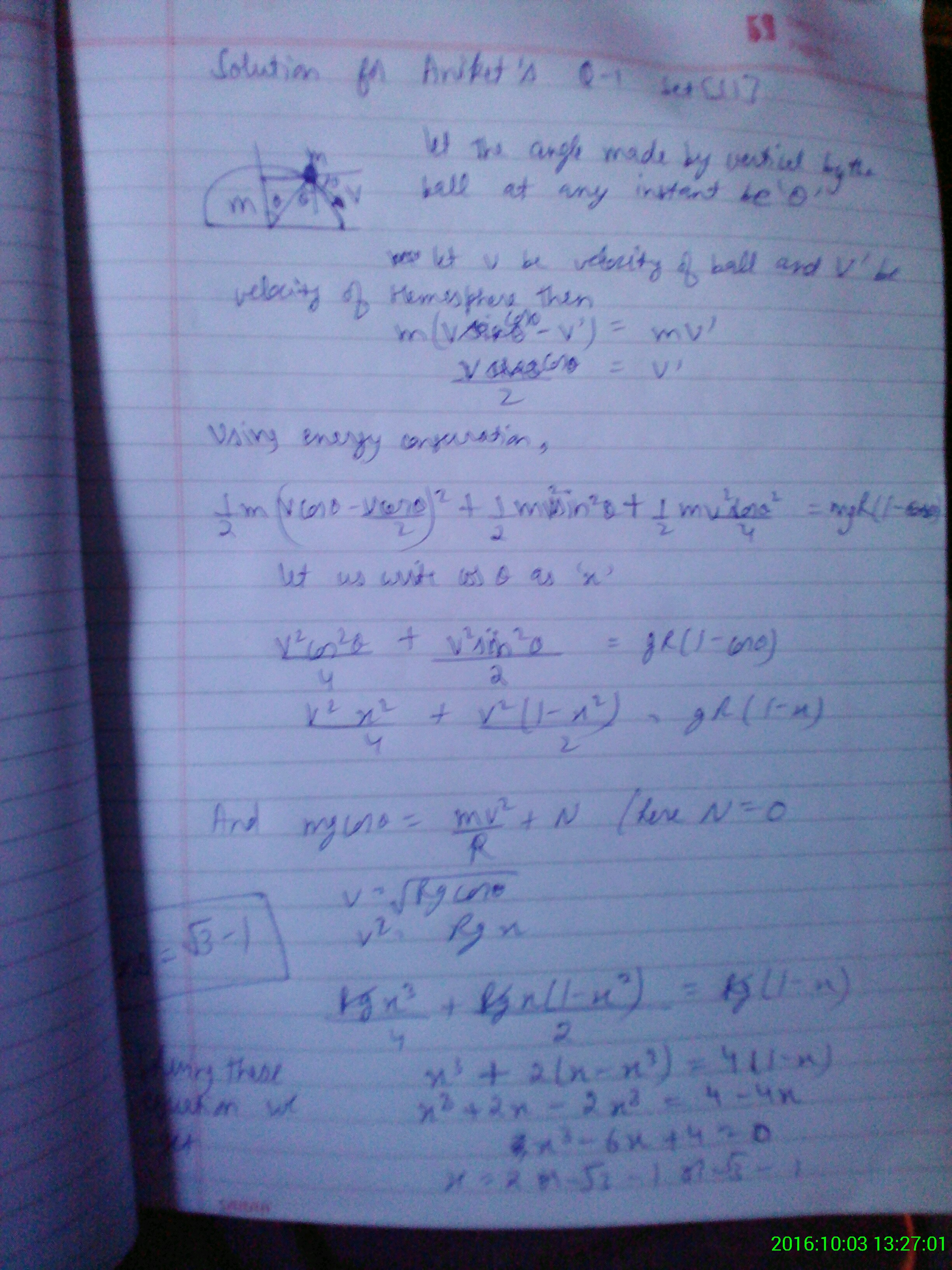Post the next problem.

- 3 years ago

That's what I wanted to say ! Q taking at least 4-5 mins This is an easy start and I hope lot more tougher q in this set ! In the second set we can go on with easy as well as hard q ! Btw yr answer is correct @shubhamdhull Post a hard q ! Hardness one step up to this :)

- 3 years ago

$Q-2\quad set-\quad II\\$ You asked for it , you got it,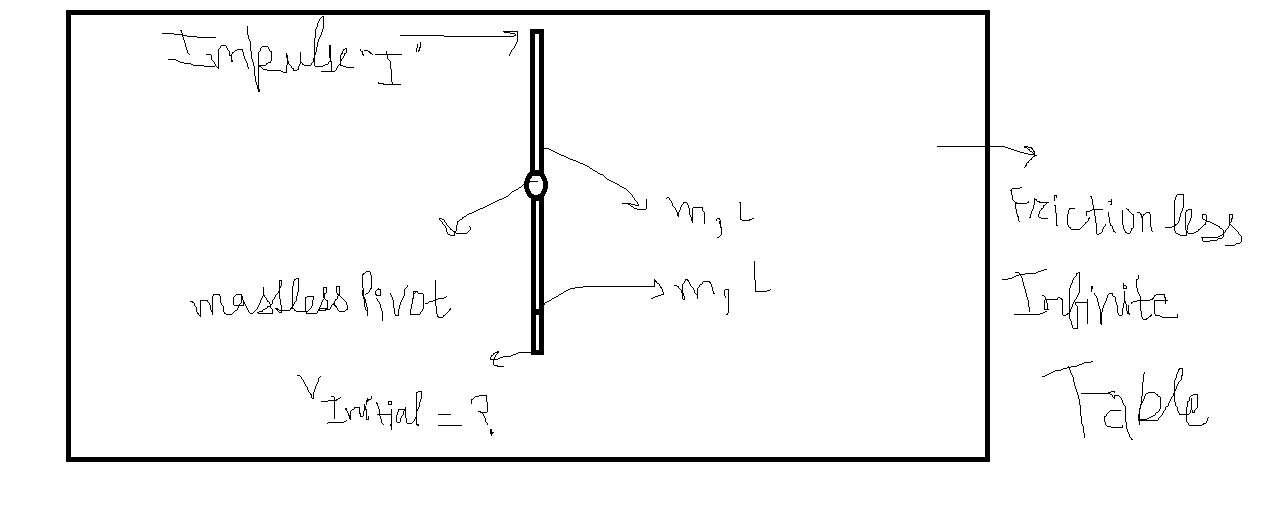My Problem ! Two similar rods are hinged by a massless pivot between them and it was given impulse " I " at its end what it the velocity of end point of other rod initially , everything is smooth and ideal . express your answer in m,L, " I "

I told u , I was in hurry to go to school! , I don't think that must be some rule!!!!! Let the hinge applies I1 towards left on the upper rod and towards right on the lower rod.

Eq goes like this I - I1 = m v1 (I + I1)L/2 = mw^2l/12 I1 = m v2 I1l/2 = mw2^2l/12

And last one

v1 - wL/2 = v2 + w2L/2

And then the thing we have to find v2 - w2L/2

On solving , we get the answer as I/2m

- 3 years ago

Yaar maine alag alag line main likha tha , sab Ek saath Ek line main aa gaya! :|

- 3 years ago

Answer I/2m correct? ( I have to go to school , I am in hurry)

- 3 years ago

ok , correct answer ,good job, now upload ur ques but keep in mind , it should be one(or two may be) level up in hardness ! a sooner reply would be appreciated and also upload a ques in set -1 as u r the solver here also ! @Aniket Sanghi

Correction : In the first set * we can go on with easy as well as hard q

- 3 years ago

A slight push *

- 3 years ago

In this second set $[Set II]$ All questions posted must be of Advance level / IPhO level .

Time provided here is 72 hours after the question is posted. Rest , the same rules go here!

- 3 years ago

I am taking part in this contest

- 2 years, 3 months ago

$Q-1$ \ i'll post the first problem so," If the kinetic energy of a particle moving on a circle varies as a function of distance travelled on the circumference of the circle as E=as^2, find the force on particle as a function of distance travelled on the circumference of the circle . "

Its quite easy.

Firstly 1/2mv^2 = 2as^2/m

As velocity vector is along the tangent to circle . differentiate this wrt s

you get

vdv/ds = 2as/m

which is nothing but a in tangential direction.

For normal accleration simply v^2/R

Now force is resultant of Normal force and tangential force :)

- 3 years ago

Bro! I guess u mean to say v^2 = 2as^2/m ( correct the typo bro! ) :)

- 3 years ago

now u post the next question !

2as rt s^2+r^2/s^2 I think

- 3 years ago

you have to show how you did it, it's a rule bro !

How to post a pic?

- 3 years ago